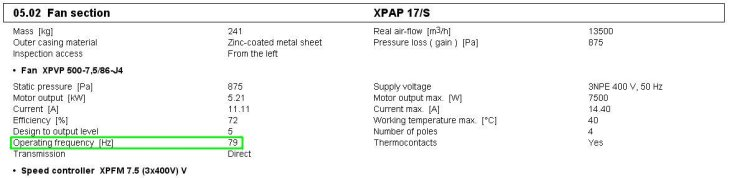Vacon 10, 100 Frequency Inverters – Working Point Frequency Setting Adjustment

Introduction

The setting of the frequency inverter to the fan working point must be performed by a trained person qualified in accordance with paragraph 6 of ČÚBP Directive No. 50/1978 Sb., and familiarized with control and parameterization of Vacon 10 and Vacon 100 frequency inverters.

Generally

The Vacon 10 and Vacon 100 frequency inverters have been preset to the maximum frequency for a given fan section type in the Remak a.s factory. This frequency corresponds to the 5th fan speed stage. Maximum frequency is stated on the fan section's plate, such as XPVP 500-7,5/86-J4. The fan working point for a given air-handling unit is included in the printed configuration output of the air-handling unit, see Figure 1. In addition to the printed output, the actual working frequency is stated on the name plate of the given section.The value of this working point (Hz) corresponds to the 5th fan speed stage. In relation to the change to the highest stage, changes to lower speed stages must also be performed.

Setting Procedure

The changes apply for the data points in which requests for frequency (stages 1 to 5) and the data point for setting the frequency to maximum are entered. For recalculation of the frequency requests, use the following formula:

Fo = PF/100 * X

FO – speed frequency for a given stage, from 1 to 5
PF – working frequency (see printed output)
X – percentage request for a required speed stage, from 1 to 5

Vacon 10 Frequency Inverter for VCB and WebClima Control Units

Enter the working point value in data point 3.8 and data point 3.2 (max. frequency) and recalculate other data points in accordance with the formula above. After recalculating by percentage from the table, the value will be equal to the frequency (Hz) instead of percents.
(Note: All frequency inverter data points must be enabled before starting the parameterization change, see P 13.1)

Data point Speed Request Percentage request "X" 3.4 1. stage 44% 3.5 2. stage 58% 3.6 3. stage 72% 3.7 4. stage 86% 3.8 5. stage 100%

Vacon 100 Frequency Inverter for VCB and WebClima Control Units
Enter the working point value in data point 3.3.14 and data point 3.3.2 (max. frequency) and recalculate other data points in accordance with the formula above. After recalculating by percentage from the table, the value will be equal to the frequency (Hz) instead of percents.

Data point Speed Request Percentage request "X" 3.4 1. stage 44% 3.5 2. stage 58% 3.6 3. stage 72% 3.7 4. stage 86% 3.8 5. stage 100%